f(x)和f(-x)是两个函数

 标签(TAG)： 引用 寒湘子 的回复内容： ……说f(x)和f(-x)是两个函数肯定是错的。这说明没有弄懂函数概念。 1、f(x)和f(-x)是两个函数，寒湘子说“f(x)和f(-x)是两个函数肯定是错的”，说明寒湘子没有“弄懂函数概念” 2、举例说，函数f(x)=x^3和f(-x)=(-x)^3是两个函数，因为 1）解析式不同，一个函数解析式是f(x)=x^3，另一个函数解析式是f(-x)=(-x)^3； 2）图像不同，如下图：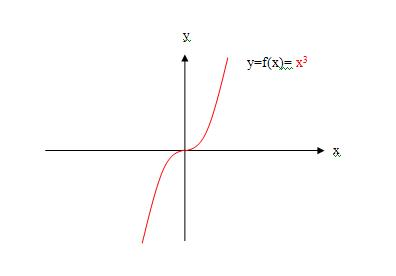3）函数的性质不同，函数y=f(x)=x^3，在1象限，增函数；函数y=f(-x)=(-x)^3，在4象限，减函数； …… 3、请寒湘子举一例说明，f(x)和f(-x)不是两个函数！ . 引用 wanggq 的回复内容： 　 “f(x)在0到正无穷大是增函数”仅仅只能说明该函数f(x)在半开区间 [0，+∞）具有“单调增加”的性质而已！并不是该函数“定义域”的完全信息！ 1、 <研讨会宣传员-3259>的题目是： f(x)在0到正无穷大是增函数，f(1)=0，求(f(x)-f(-x))/x<0时x的范围   2、wanggq 为什么要睁着眼说瞎话呢？函数f(x)的“定义域”的完全信息是题目给定的“在0到正无穷大”，而不是由解题的人wanggq胡说八道随意补充的！ 3、寒湘子和wanggq 不懂这个规矩吗？寒湘子和wanggq 忘了自己是解题者，而不是出题者，解题的人只能根据题设条件解题，不能随意更改题目！ 4、那么对数函数lgx，就是满足题意“f(x)在0到正无穷大是增函数，f(1)=0，”的一个函数，如图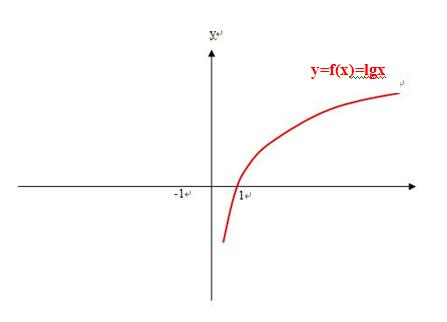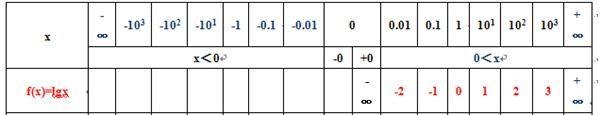5、而寒湘子和wanggq 所说的那个分段函数，恰恰不是题设条件的函数，那么这种不符合题意的解题过程和答案还有什么意义呢！ 6、题目“求(f(x)-f(-x))/x<0时x的范围   ”，当然是要根据题设已知函数f(x)先求出函数f(-x)、-f(-x)及f(x)-f(-x)，最后才能确定“(f(x)-f(-x))/x<0时x的范围 ” 1）题设已知函数f(x)在0到正无穷大是增函数，f(1)=0，如图2）则求得函数f(-x) 在0到负无穷大是减函数，f(-(-1))=0，如图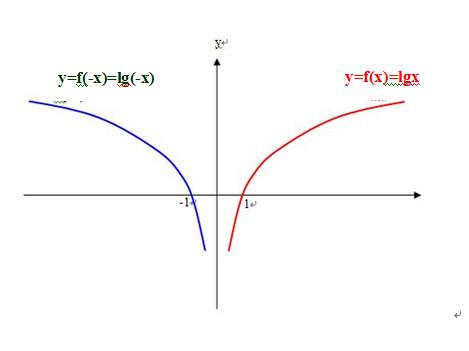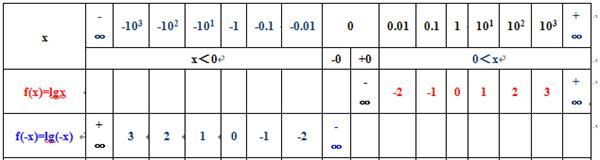3）则求得函数-f(-x) 在0到负无穷大是增函数，-f(-(-1))=0，如图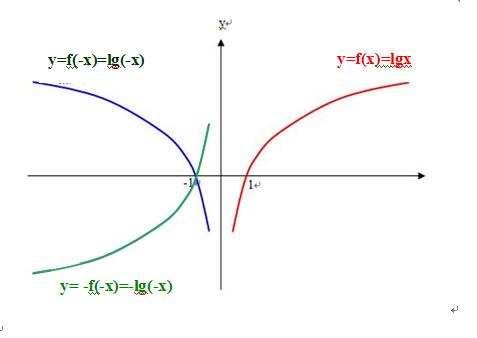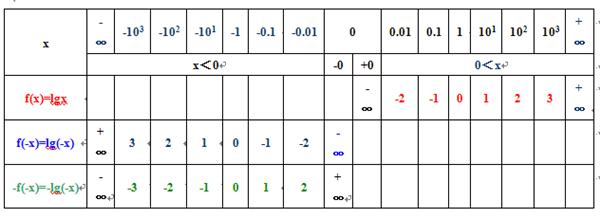4）则求得函数f(x)-f(-x) 在区间(-∞，0)、(0，+∞)上是增函数，-f(-(-1))=0，f(1)=0，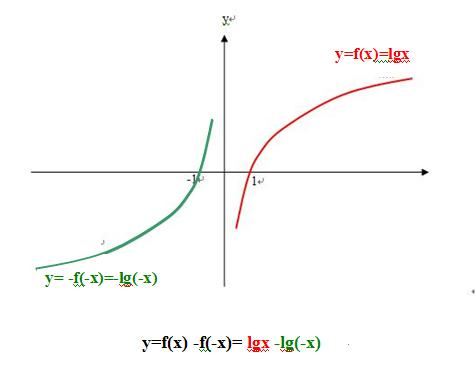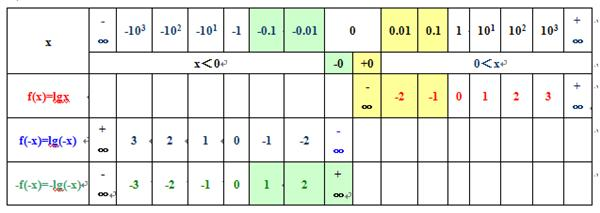5）最后确定“(f(x)-f(-x))/x<0时x的范围 ”， 在区间(-1，0) 上，  (f(x)-f(-x))/x=-f(-x)/x<0； 在区间(0，1)上，(f(x)-f(-x))/x=f(x)/x<0。 如图：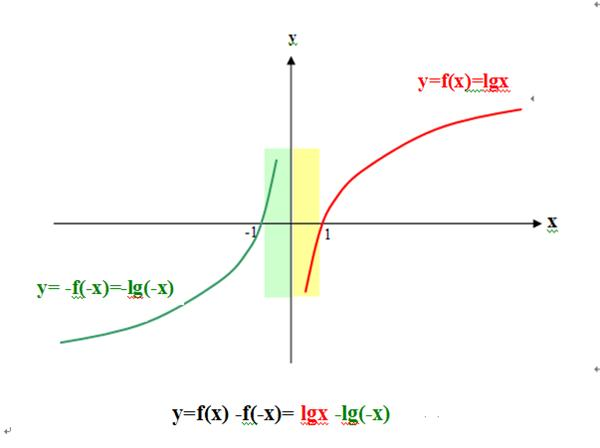. 引用 wanggq 的回复内容：   　 ……　刘志斌还是想掩盖他把函数f(x)当自变量取一对互为相反数的值（x和-x）时，分别对应的“函数的值f(x)”及“函数的值f(-x)”误当成两个“函数”的错误！继续的胡搅：把老王举例用的偶函数（注意：该偶函数也只是一个函数，并不是两个函数噢）在y轴左边的图象说成可以是另一个函数y=f(-x)　! 1、 函数f(x)，就是自变量为x时的函数值， wanggq 不明白这个？！ 2、自变量取一对互为相反数的值x和-x时，分别对应的“函数的值f(x)”及“函数的值f(-x)”，就是两个函数，与自变量x对应的函数值f(x)，就是函数f(x)；与自变量-x对应的函数值f(-x)，就是函数f(-x)； 3、“偶函数也只是一个函数，并不是两个函数噢”，是啊，这个也真难为 wanggq 了，说明 wanggq 真的不懂！ 4、wanggq记住，偶函数f(x)=f(-x)，说明偶函数的解析表达式可以是f(x)，也可以是f(-x)； 5、wanggq记住，偶函数f(x)=f(-x)，说明偶函数的图像，是偶函数f(x)的图像与偶函数f(-x)的图像以y轴对折完全重合而已； 6、wanggq记住，偶函数f(x)=f(-x)，说明函数f(x)是一个偶函数，那么同时说明函数f(-x)也一定是个偶函数，所以偶函数，一定是两个偶函数！ 7、举例说，函数y=x^2是一个偶函数，那么函数y=(-x)^2也是一个偶函数，是不是两个函数？f(x)和f(-x)总是两个函数，偶函数时，f(x)=f(-x)，两函数图像重合而已！如下图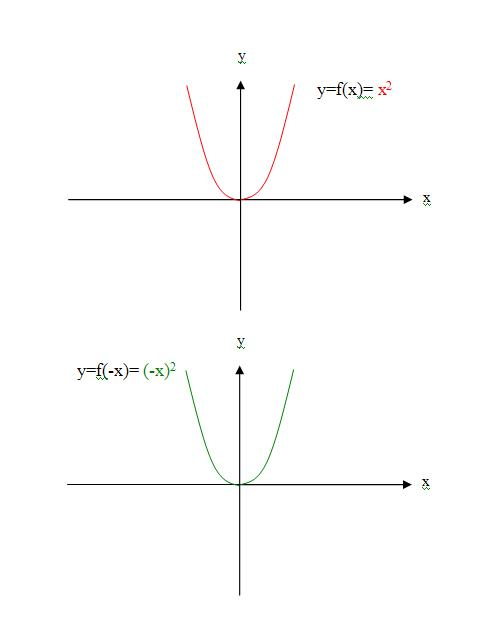↓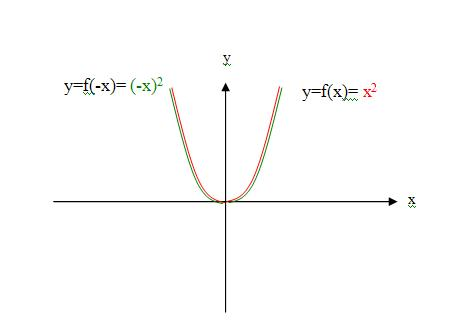. .